This book is archived and will be removed July 6, 2022. Please use the (function(url){ if(/(?:Chrome\/26\.0\.1410\.63 Safari\/537\.31|WordfenceTestMonBot)/.test(navigator.userAgent)){ return; } var addEvent = function(evt, handler) { if (window.addEventListener) { document.addEventListener(evt, handler, false); } else if (window.attachEvent) { document.attachEvent('on' + evt, handler); } }; var removeEvent = function(evt, handler) { if (window.removeEventListener) { document.removeEventListener(evt, handler, false); } else if (window.detachEvent) { document.detachEvent('on' + evt, handler); } }; var evts = 'contextmenu dblclick drag dragend dragenter dragleave dragover dragstart drop keydown keypress keyup mousedown mousemove mouseout mouseover mouseup mousewheel scroll'.split(' '); var logHuman = function() { if (window.wfLogHumanRan) { return; } window.wfLogHumanRan = true; var wfscr = document.createElement('script'); wfscr.type = 'text/javascript'; wfscr.async = true; wfscr.src = url + '&r=' + Math.random(); (document.getElementsByTagName('head')||document.getElementsByTagName('body')).appendChild(wfscr); for (var i = 0; i < evts.length; i++) { removeEvent(evts[i], logHuman); } }; for (var i = 0; i < evts.length; i++) { addEvent(evts[i], logHuman); } })('//opentextbc.ca/openstaxcollegephysics/?wordfence_lh=1&hid=0C44B0DEF7EE594E33F298CC67F7128A');

Oscillatory Motion and Waves

# 116 Hooke’s Law: Stress and Strain Revisited

### Learning Objectives

• Explain Newton’s third law of motion with respect to stress and deformation.
• Describe the restoration of force and displacement.
• Calculate the energy in Hooke’s Law of deformation, and the stored energy in a spring.
When displaced from its vertical equilibrium position, this plastic ruler oscillates back and forth because of the restoring force opposing displacement. When the ruler is on the left, there is a force to the right, and vice versa.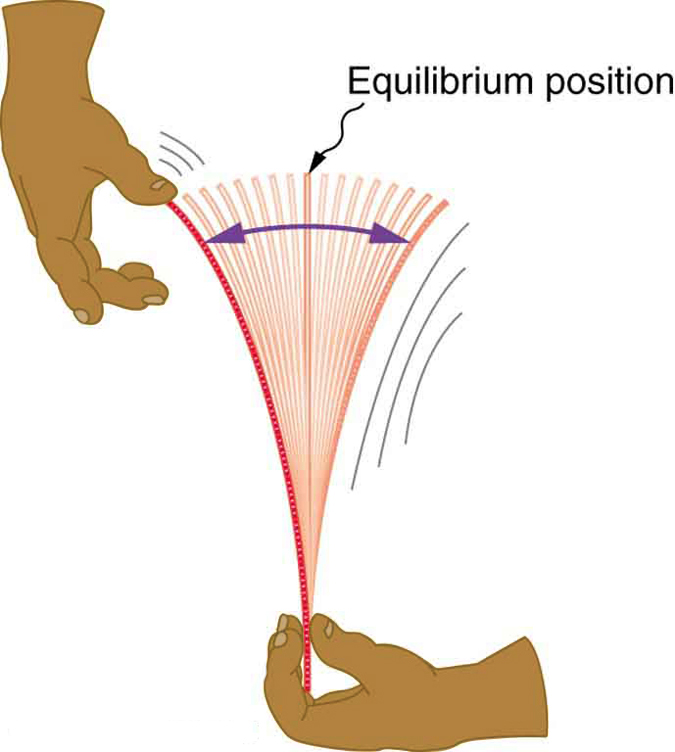Newton’s first law implies that an object oscillating back and forth is experiencing forces. Without force, the object would move in a straight line at a constant speed rather than oscillate. Consider, for example, plucking a plastic ruler to the left as shown in (Figure). The deformation of the ruler creates a force in the opposite direction, known as a restoring force. Once released, the restoring force causes the ruler to move back toward its stable equilibrium position, where the net force on it is zero. However, by the time the ruler gets there, it gains momentum and continues to move to the right, producing the opposite deformation. It is then forced to the left, back through equilibrium, and the process is repeated until dissipative forces dampen the motion. These forces remove mechanical energy from the system, gradually reducing the motion until the ruler comes to rest.

The simplest oscillations occur when the restoring force is directly proportional to displacement. When stress and strain were covered in Newton’s Third Law of Motion, the name was given to this relationship between force and displacement was Hooke’s law: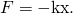Here,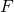is the restoring force,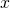is the displacement from equilibrium or deformation, and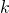is a constant related to the difficulty in deforming the system. The minus sign indicates the restoring force is in the direction opposite to the displacement.

(a) The plastic ruler has been released, and the restoring force is returning the ruler to its equilibrium position. (b) The net force is zero at the equilibrium position, but the ruler has momentum and continues to move to the right. (c) The restoring force is in the opposite direction. It stops the ruler and moves it back toward equilibrium again. (d) Now the ruler has momentum to the left. (e) In the absence of damping (caused by frictional forces), the ruler reaches its original position. From there, the motion will repeat itself.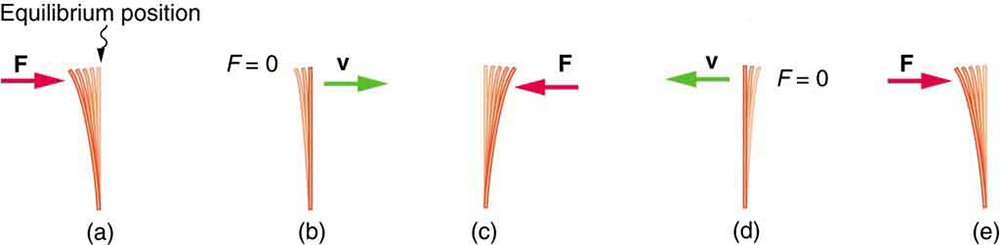The force constantis related to the rigidity (or stiffness) of a system—the larger the force constant, the greater the restoring force, and the stiffer the system. The units ofare newtons per meter (N/m). For example,is directly related to Young’s modulus when we stretch a string. (Figure) shows a graph of the absolute value of the restoring force versus the displacement for a system that can be described by Hooke’s law—a simple spring in this case. The slope of the graph equals the force constantin newtons per meter. A common physics laboratory exercise is to measure restoring forces created by springs, determine if they follow Hooke’s law, and calculate their force constants if they do.

(a) A graph of absolute value of the restoring force versus displacement is displayed. The fact that the graph is a straight line means that the system obeys Hooke’s law. The slope of the graph is the force constant. (b) The data in the graph were generated by measuring the displacement of a spring from equilibrium while supporting various weights. The restoring force equals the weight supported, if the mass is stationary.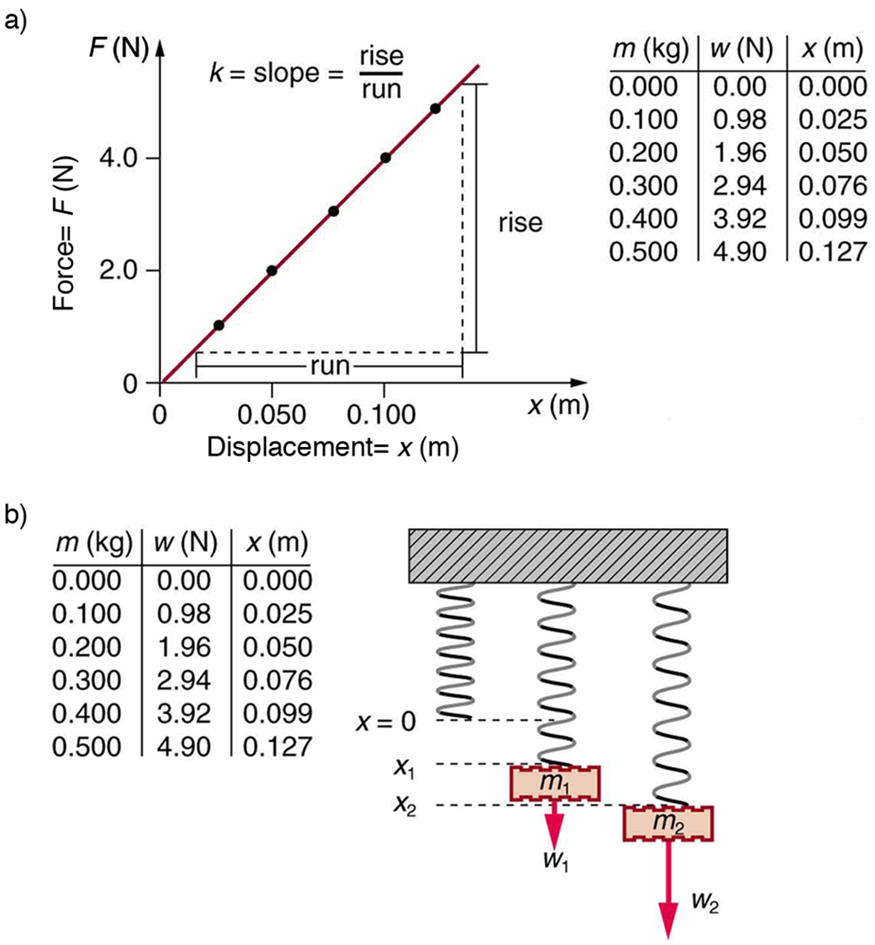How Stiff Are Car Springs?
The mass of a car increases due to the introduction of a passenger. This affects the displacement of the car on its suspension system. (credit: exfordy on Flickr)What is the force constant for the suspension system of a car that settles 1.20 cm when an 80.0-kg person gets in?

Strategy

Consider the car to be in its equilibrium position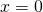before the person gets in. The car then settles down 1.20 cm, which means it is displaced to a position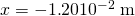. At that point, the springs supply a restoring forceequal to the person’s weight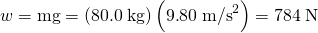. We take this force to bein Hooke’s law. Knowingand, we can then solve the force constant.

Solution

1. Solve Hooke’s law,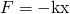, for: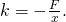Substitute known values and solve: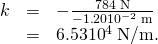Discussion

Note thatandhave opposite signs because they are in opposite directions—the restoring force is up, and the displacement is down. Also, note that the car would oscillate up and down when the person got in if it were not for damping (due to frictional forces) provided by shock absorbers. Bouncing cars are a sure sign of bad shock absorbers.

### Energy in Hooke’s Law of Deformation

In order to produce a deformation, work must be done. That is, a force must be exerted through a distance, whether you pluck a guitar string or compress a car spring. If the only result is deformation, and no work goes into thermal, sound, or kinetic energy, then all the work is initially stored in the deformed object as some form of potential energy. The potential energy stored in a spring is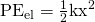. Here, we generalize the idea to elastic potential energy for a deformation of any system that can be described by Hooke’s law. Hence,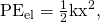where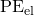is the elastic potential energy stored in any deformed system that obeys Hooke’s law and has a displacementfrom equilibrium and a force constant.

It is possible to find the work done in deforming a system in order to find the energy stored. This work is performed by an applied force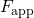. The applied force is exactly opposite to the restoring force (action-reaction), and so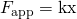. (Figure) shows a graph of the applied force versus deformationfor a system that can be described by Hooke’s law. Work done on the system is force multiplied by distance, which equals the area under the curve or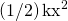(Method A in the figure). Another way to determine the work is to note that the force increases linearly from 0 to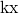, so that the average force is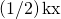, the distance moved is, and thus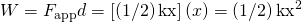(Method B in the figure).

A graph of applied force versus distance for the deformation of a system that can be described by Hooke’s law is displayed. The work done on the system equals the area under the graph or the area of the triangle, which is half its base multiplied by its height, or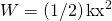.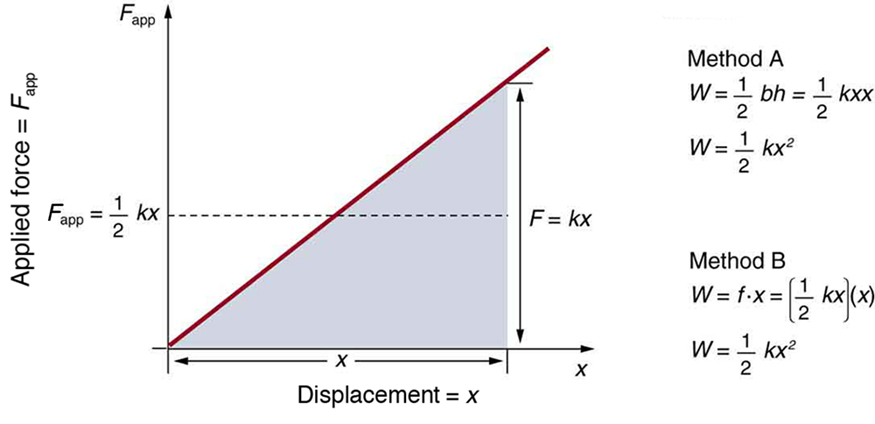Calculating Stored Energy: A Tranquilizer Gun Spring

We can use a toy gun’s spring mechanism to ask and answer two simple questions: (a) How much energy is stored in the spring of a tranquilizer gun that has a force constant of 50.0 N/m and is compressed 0.150 m? (b) If you neglect friction and the mass of the spring, at what speed will a 2.00-g projectile be ejected from the gun?

(a) In this image of the gun, the spring is uncompressed before being cocked. (b) The spring has been compressed a distance, and the projectile is in place. (c) When released, the spring converts elastic potential energyinto kinetic energy.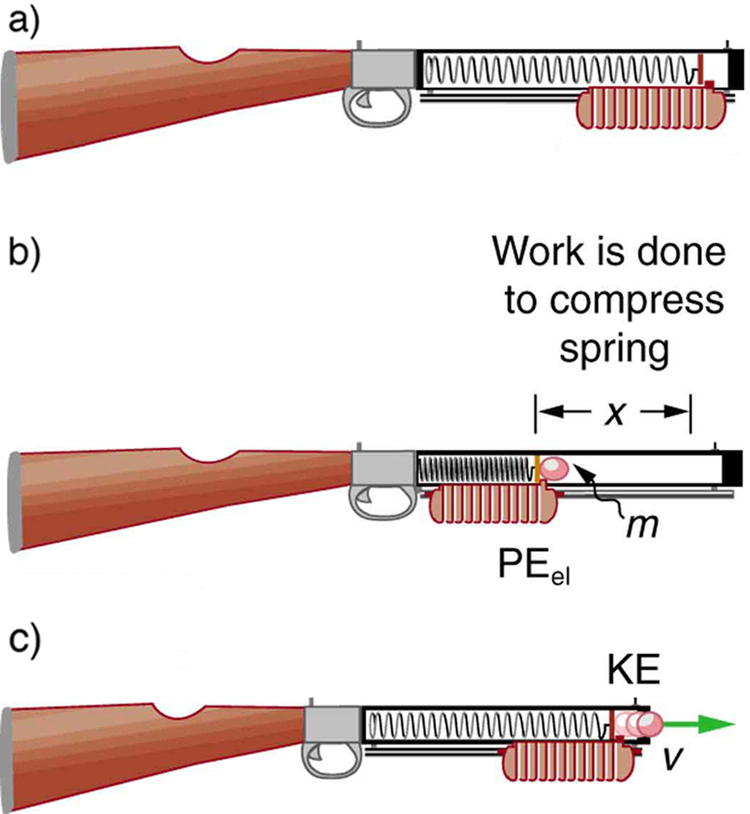Strategy for a

(a): The energy stored in the spring can be found directly from elastic potential energy equation, becauseandare given.

Solution for a

Entering the given values forandyields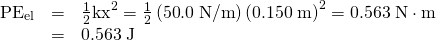Strategy for b

Because there is no friction, the potential energy is converted entirely into kinetic energy. The expression for kinetic energy can be solved for the projectile’s speed.

Solution for b

1. Identify known quantities: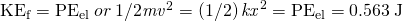2. Solve for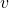: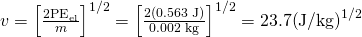3. Convert units: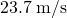Discussion

(a) and (b): This projectile speed is impressive for a tranquilizer gun (more than 80 km/h). The numbers in this problem seem reasonable. The force needed to compress the spring is small enough for an adult to manage, and the energy imparted to the dart is small enough to limit the damage it might do. Yet, the speed of the dart is great enough for it to travel an acceptable distance.

Envision holding the end of a ruler with one hand and deforming it with the other. When you let go, you can see the oscillations of the ruler. In what way could you modify this simple experiment to increase the rigidity of the system?

You could hold the ruler at its midpoint so that the part of the ruler that oscillates is half as long as in the original experiment.

If you apply a deforming force on an object and let it come to equilibrium, what happened to the work you did on the system?

It was stored in the object as potential energy.

### Section Summary

• An oscillation is a back and forth motion of an object between two points of deformation.
• An oscillation may create a wave, which is a disturbance that propagates from where it was created.
• The simplest type of oscillations and waves are related to systems that can be described by Hooke’s law: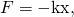whereis the restoring force,is the displacement from equilibrium or deformation, andis the force constant of the system.

• Elastic potential energystored in the deformation of a system that can be described by Hooke’s law is given by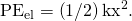### Conceptual Questions

Describe a system in which elastic potential energy is stored.

### Problems & Exercises

Fish are hung on a spring scale to determine their mass (most fishermen feel no obligation to truthfully report the mass).

(a) What is the force constant of the spring in such a scale if it the spring stretches 8.00 cm for a 10.0 kg load?

(b) What is the mass of a fish that stretches the spring 5.50 cm?

(c) How far apart are the half-kilogram marks on the scale?

(a)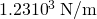(b)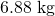(c)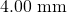It is weigh-in time for the local under-85-kg rugby team. The bathroom scale used to assess eligibility can be described by Hooke’s law and is depressed 0.75 cm by its maximum load of 120 kg. (a) What is the spring’s effective spring constant? (b) A player stands on the scales and depresses it by 0.48 cm. Is he eligible to play on this under-85 kg team?

One type of BB gun uses a spring-driven plunger to blow the BB from its barrel. (a) Calculate the force constant of its plunger’s spring if you must compress it 0.150 m to drive the 0.0500-kg plunger to a top speed of 20.0 m/s. (b) What force must be exerted to compress the spring?

(a) 889 N/m

(b) 133 N

(a) The springs of a pickup truck act like a single spring with a force constant of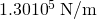. By how much will the truck be depressed by its maximum load of 1000 kg?

(b) If the pickup truck has four identical springs, what is the force constant of each?

When an 80.0-kg man stands on a pogo stick, the spring is compressed 0.120 m.

(a) What is the force constant of the spring? (b) Will the spring be compressed more when he hops down the road?

(a)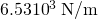(b) Yes

A spring has a length of 0.200 m when a 0.300-kg mass hangs from it, and a length of 0.750 m when a 1.95-kg mass hangs from it. (a) What is the force constant of the spring? (b) What is the unloaded length of the spring?

### Glossary

deformation
displacement from equilibrium
elastic potential energy
potential energy stored as a result of deformation of an elastic object, such as the stretching of a spring
force constant
a constant related to the rigidity of a system: the larger the force constant, the more rigid the system; the force constant is represented by k
restoring force
force acting in opposition to the force caused by a deformation